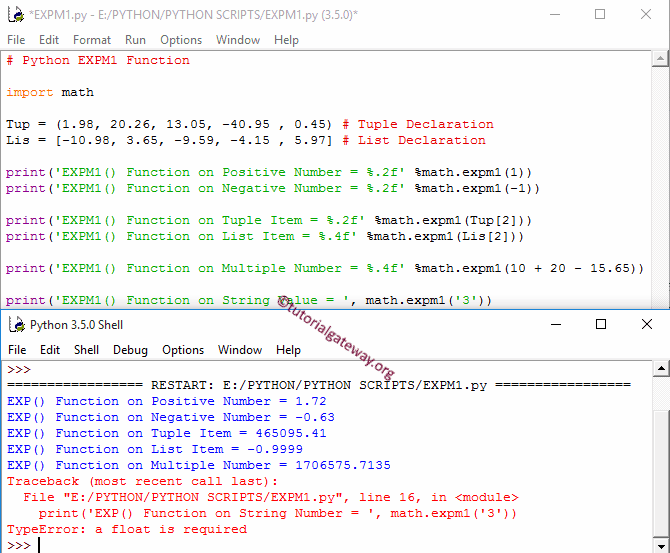# Python expm1

The Python expm1 math function is to calculate the power of E (Where E is Euler’s number approximately equal to 2.71828) and subtracts One from it. In this section, we discuss how to use expm1 function with an example.

The syntax of the expm1 Function is

`math.expm1(number);`

Or we can simply say math.exp(number) – 1. Please refer the exp function for further reference.

Number: It can be a number or a valid numerical expression.

• If the number argument is a positive or negative number, it returns the output.
• If the number argument is not a number, the function return TypeError.

## Python expm1 Function Example

The expm1 Function calculates the power of Euler’s number E and subtracts One from it. In this example, we use this one to find the power of e of different data types.

```import math

Tup = (1.98, 20.26, 13.05, -40.95 , 0.45) # Tuple Declaration
Lis = [-10.98, 3.65, -9.59, -4.15 , 5.97] # List Declaration

print('EXPM1() Function on Positive Number = %.2f' %math.expm1(1))
print('EXPM1() Function on Negative Number = %.2f' %math.expm1(-1))

print('EXPM1() Function on Tuple Item = %.2f' %math.expm1(Tup))
print('EXPM1() Function on List Item = %.4f' %math.expm1(Lis))

print('EXPM1() Function on Multiple Number = %.4f' %math.expm1(10 + 20 - 15.65))

print('EXPM1() Function on String Value = ', math.expm1('3'))```In the Last statement, We used it on the String value. And as we said before, it returns TypeError as output. Please refer to Tuple, List, and Math functions in Python.